# const - JS | lectureHow JavaScript Works Behind the Scenes

Primitives VS Objects (Primitive VS Reference Types)

As we move towards the end of this section, we need to learn about the big difference between the way primitive types and objects are stored in memory.

This is a very practical aspect and one that causes a lot of confusion in beginners. Let's start by writing some code this time to understand the confusion so that I can then show you how it all works behind the scenes.

Right now, I'm not going to explain how the code we will write now works. I will show you the source of confusion and explain it later. Let's start with a simple example with primitives. Remember that primitives are numbers, strings, booleans, etc.

script.js
``````let ag = 30;
let oldAge = age;
age = 31;

console.log(age); // Result ==> 31
console.log(oldAge); // Result ==> 30``````

If we now reload our browser, they should be pretty much what we expect at this point.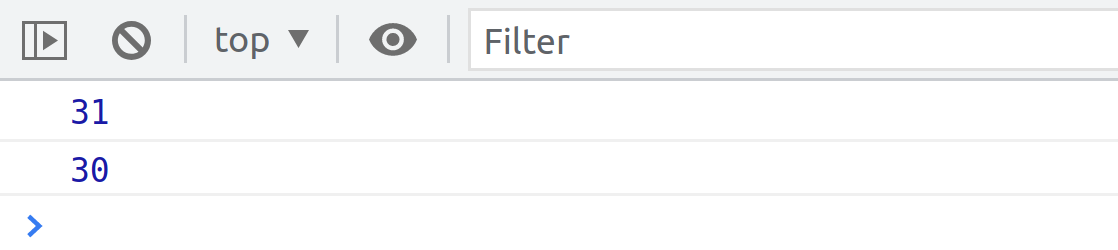The above result shows that the current age is 31 because we changed the original one from 30 to 31. But the old age is still 30, and that's because, on line 2, we set it to age, which was still 30. So changing the age on line 3 from 30 to 31 did not affect the old age variable again because, again, on line 2, age was still 30. All right, so here, hopefully, there is not much confusion. Everything works just as expected.

Now let's create another scenario that has an object.

script.js
``````const john = {
name: 'John',
age: 30,
};``````

Now let's copy this object because let's say that John has a friend who's also called John. And so, instead of creating a brand new object from scratch, let's ust copy the `john` object.

script.js
``const friend = john;``

Both the name and the age are the same, but let's say that they have different ages. Let's change the age of John's friend

script.js
``friend.age = 28``

Let's now see both objects in the console.

script.js
``````console.log('Friend: ', friend)
console.log('John: ', john)``````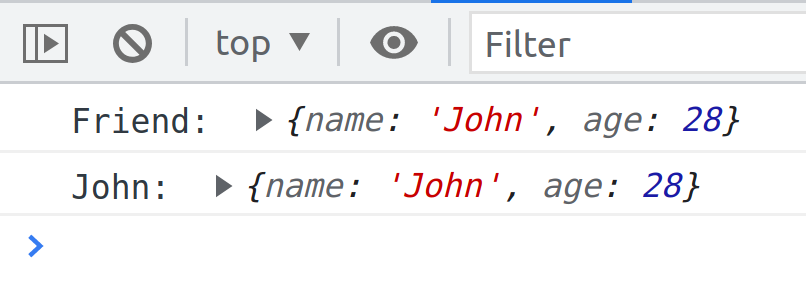From above, we see that both `john` and `friend` have the age of 28. That looks a little bit strange because all we did was change the friend's age. Nowhere in the code, do we have `john.age = 28`, but still, age in the `john` object is also 28.

That is what I mean by a source of confusion. In this lecture, let's now find out why it works this way.

Before we can understand the code we just wrote, we need to review some basics here. First, we need to remember JavaScript primitive data types: number, string, Boolean, undefined, null, symbol, and BigInt.

Then everything else are objects. So objects created with the object literal, arrays, and even functions are all objects.

Now, when we're talking about memory and memory management, it's usual to call primitives, primitive types, and objects reference types because of the different ways in which they are stored in memory.

Next, we need to remember about the JavaScript engine. The engine has two components, the call stack, where functions are executed, and the heap, where objects are stored in memory.

All our objects, or in other words, reference types, will get stored right in the memory heap. When we first talked about the engine, I mentioned that, but now you will finally learn how that works.

On the other hand, primitives or primitive types are stored in the call stack. And with that, I mean that primitive types are stored in the execution contexts in which they are declared. But for the sake of simplicity, let's ignore that detail now and simply say that primitive types are stored in the call stack because that's where execution context runs.

All right, but now how does all that actually work? And why did our code example earlier behave in that weird way? Well, let's find out.

Below we have the two code examples from earlier, as well as the engine with call stack and heap. Let's start by looking at the example of primitive values.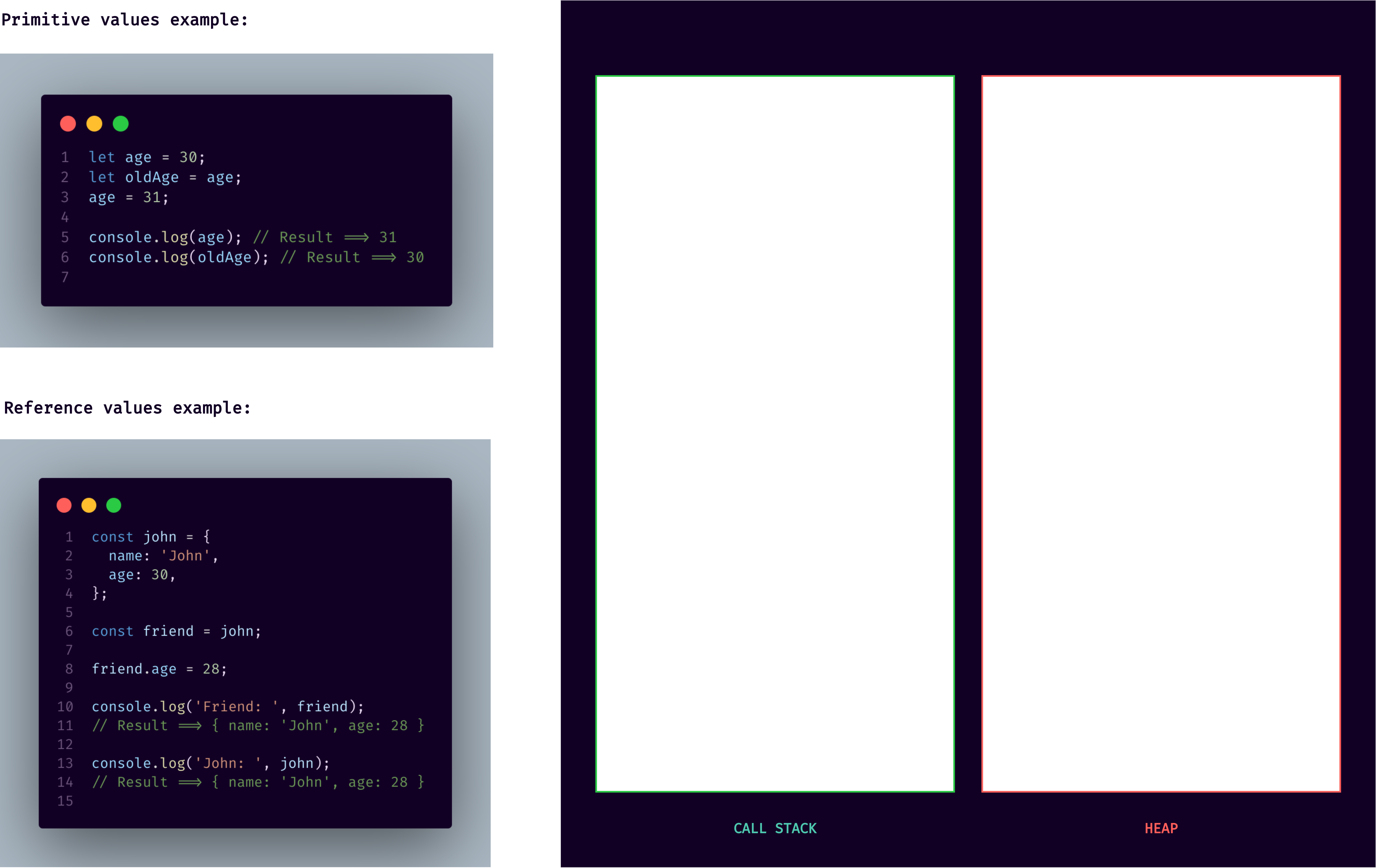When we declare a variable like `age` equals 30, what actually happens inside the JavaScript engine and the computer's memory? First, JavaScript will create a so-called unique identifier with the variable name. Then a piece of memory will be allocated with a certain address, so `0237` in this example, and finally, the value will be stored in memory at the specified address. So, in this case, the value 30 will be specified at memory address 0237. And remember, this all happens in a call stack where primitive values are stored.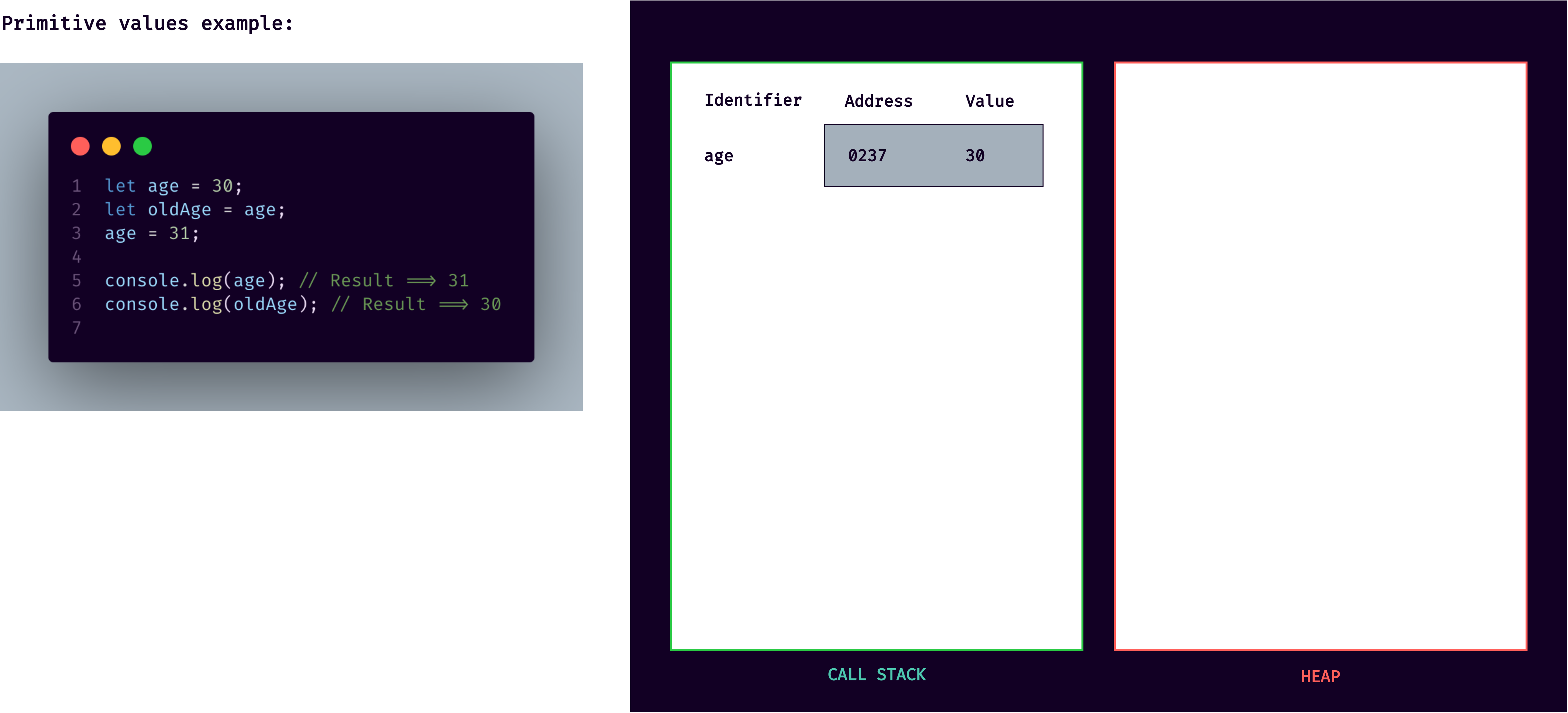Now what's extremely important to understand here is that the identifier actually points to the address and not the value itself. So we would say that the age variable is equal to 30, but in fact, age is equal to the memory address 0237, which holds the value of 30. This subtle distinction is very important to keep in mind.

In the next line, we declare old age to be equal to age. Knowing that a variable actually holds a memory address, what should old age look like? It will simply point to the same memory address as the age variable. It will look like old age, is simply 30 as well.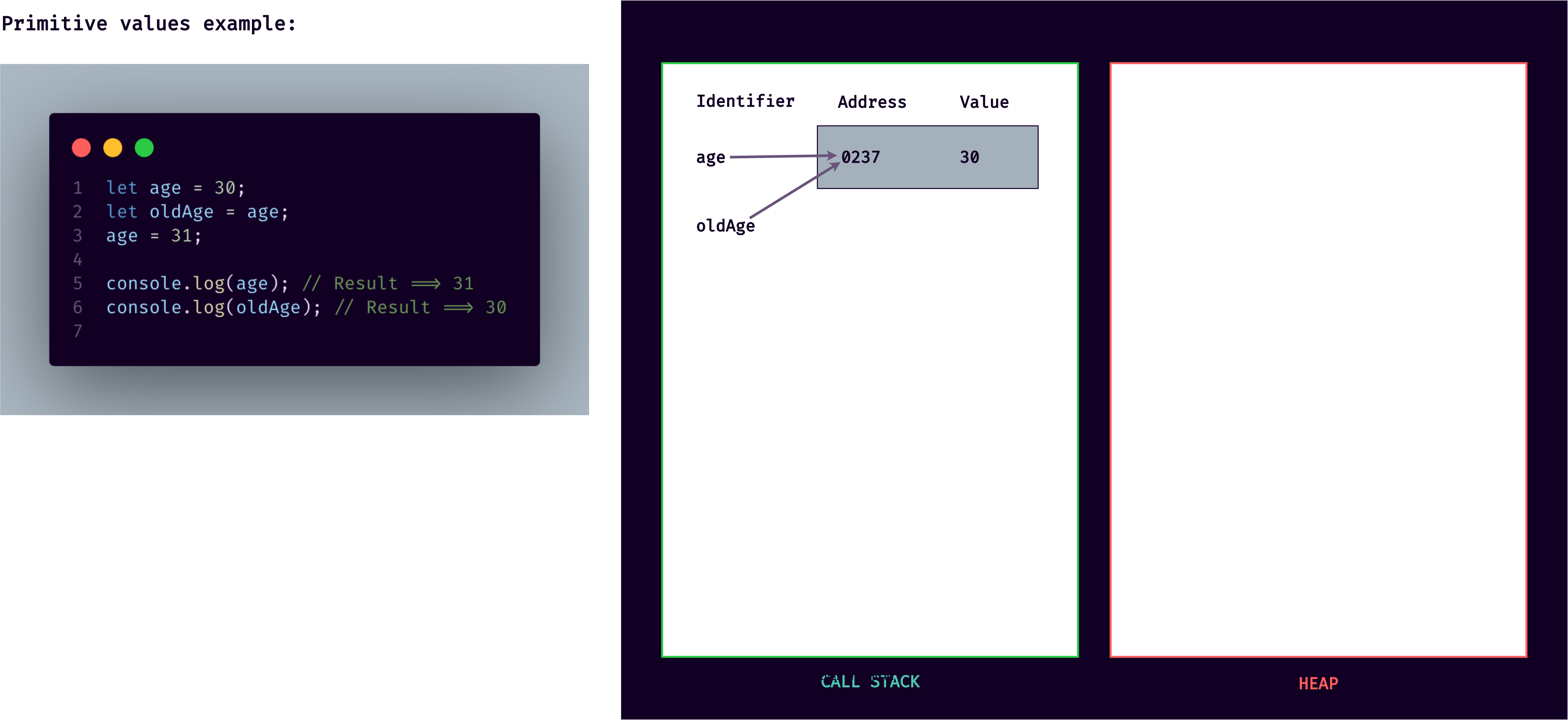Great, but now in the next line, we set age to 31. What will happen then? The value at address 0237 will certainly not become 31 because that would change old age as well, since they both point to the same memory address. That would make no sense at all. Also, the value at a certain memory address is immutable, or in other words, it cannot be changed. So instead, what's going to happen here is that a new piece of memory is allocated.

It's created, and the age identifier now points to the new address, which holds the new value of 31. That's why when we log both variables to the console, in the end, they both return exactly the values that we expect.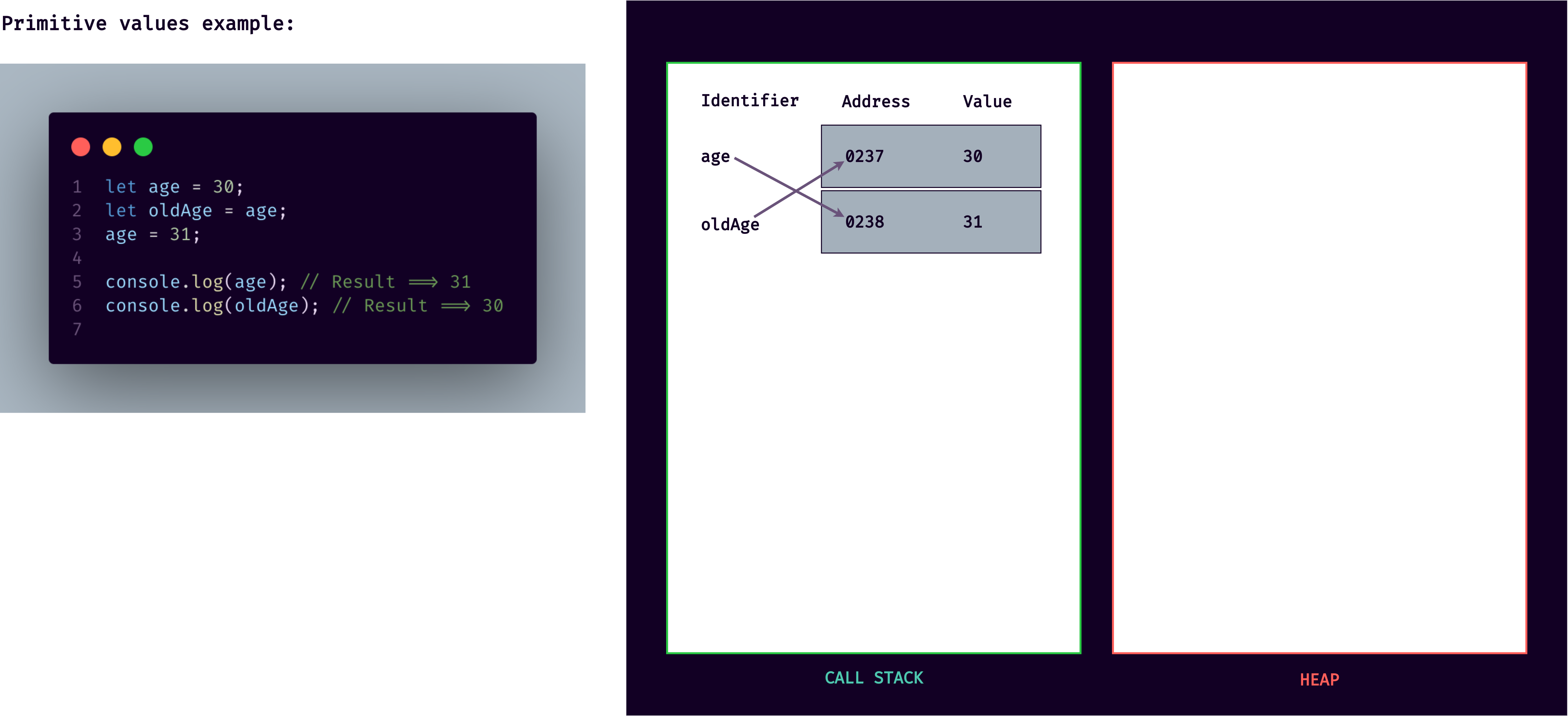With reference values, things work a bit differently, which is why the below example gave us that unexpected, weird behavior earlier in practice.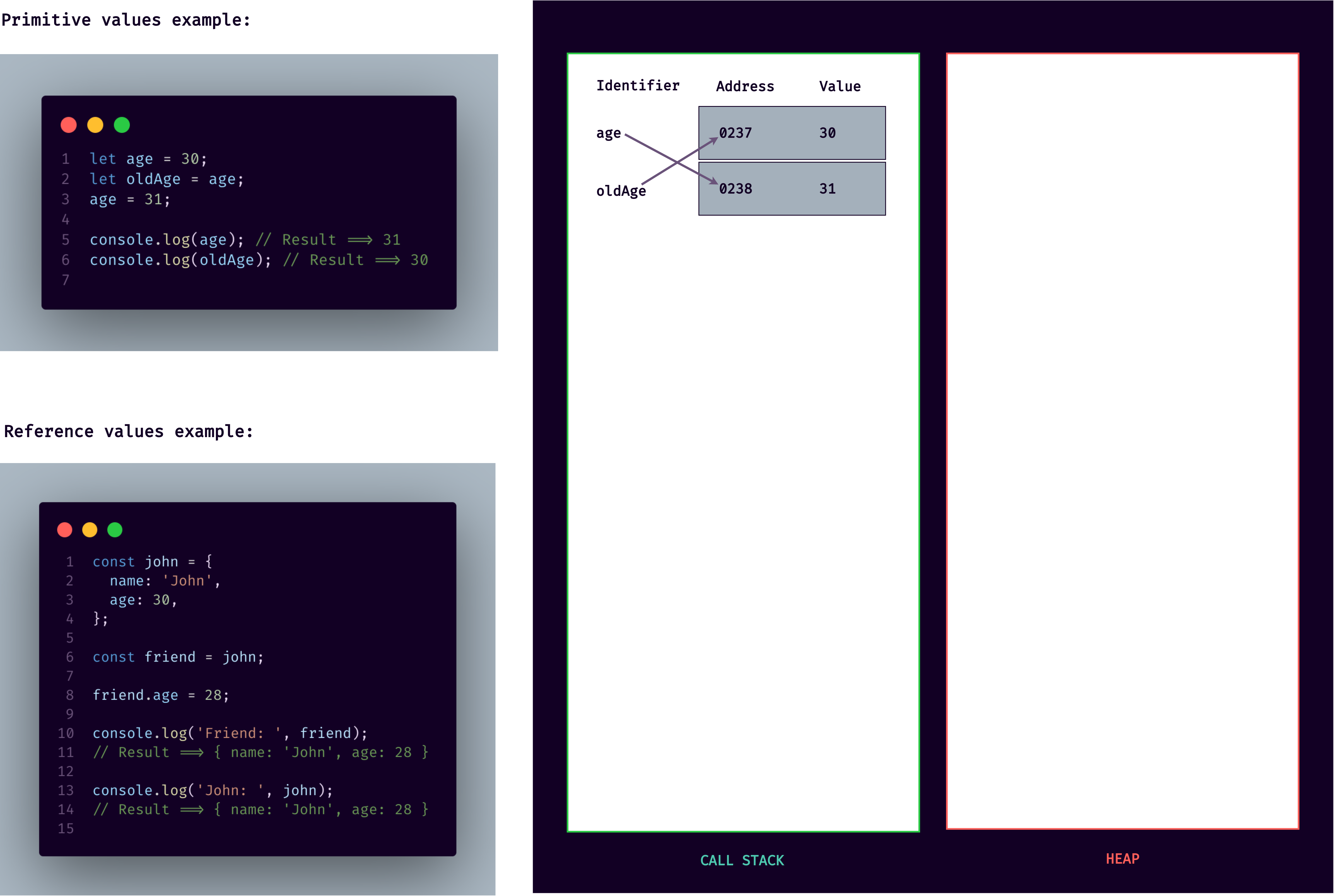So what's the origin of this weird, unexpected result ? When a new object is created, such as the `john` object, it is stored in the heap. And just like before, there is a memory address and then the value itself.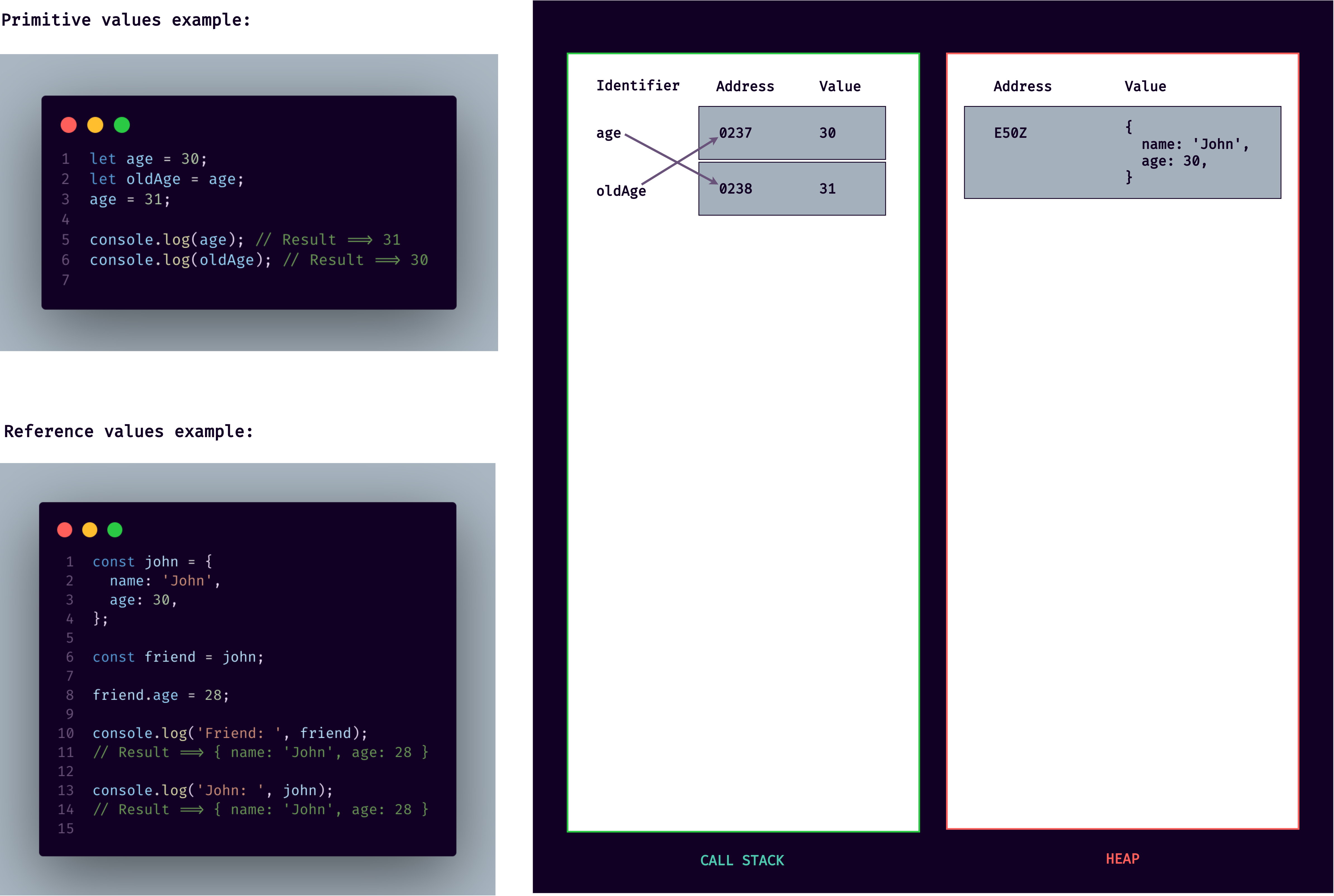In the case of reference values like the `john` object, the `john` identifier does not point directly to the newly created memory address in the heap. In this example, E50Z, instead, it will point to a new piece of memory that's created in the stack. And this new piece of memory will then point to the object that's in the heap by using the memory address as its value.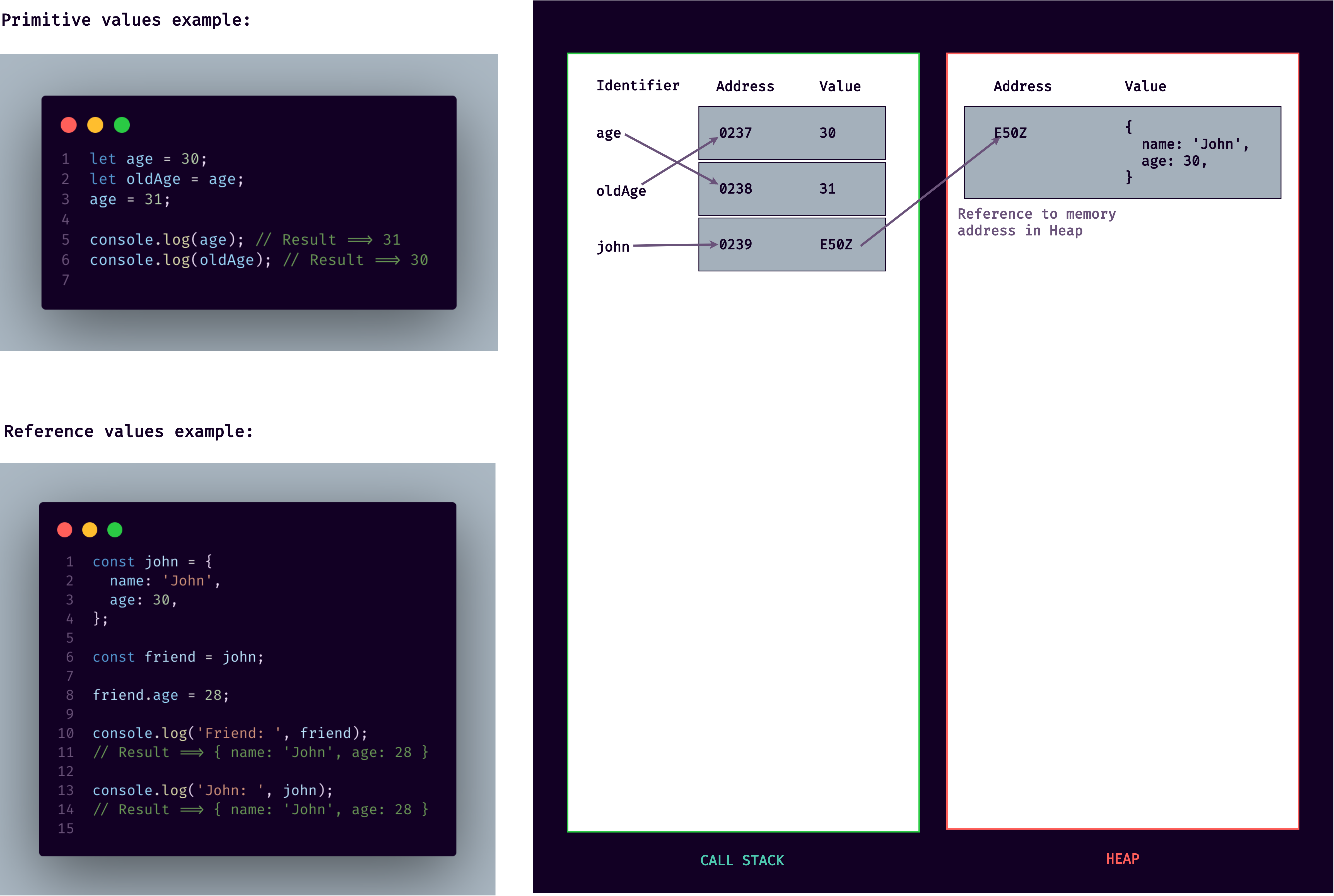In other words, the piece of memory in the call stack has a reference to the piece of memory in the heap, which holds our `john` object. That's the reason why we call objects reference types in this context.

Again, when we declare a variable as an object, an identifier is created, which points to a piece of memory in the stack, which in turn points to a piece of memory in the heap. And that is where the object is actually stored. It works this way because objects might be too large to be stored in the stack. Instead, they are stored in the heap, which is like an almost unlimited memory pool. The stack just keeps a reference to where the object is actually stored in the heap so that it can find it whenever necessary.

Now, moving on in the code, we create a new variable called `friend` that we set equal to the `john` object. Just like with primitive values, the `friend` identifier will point to the same memory address as the `john` identifier. And again, that address contains the reference, which then points to the object itself. And like this, the `friend` object is now essentially the same as the `john` object.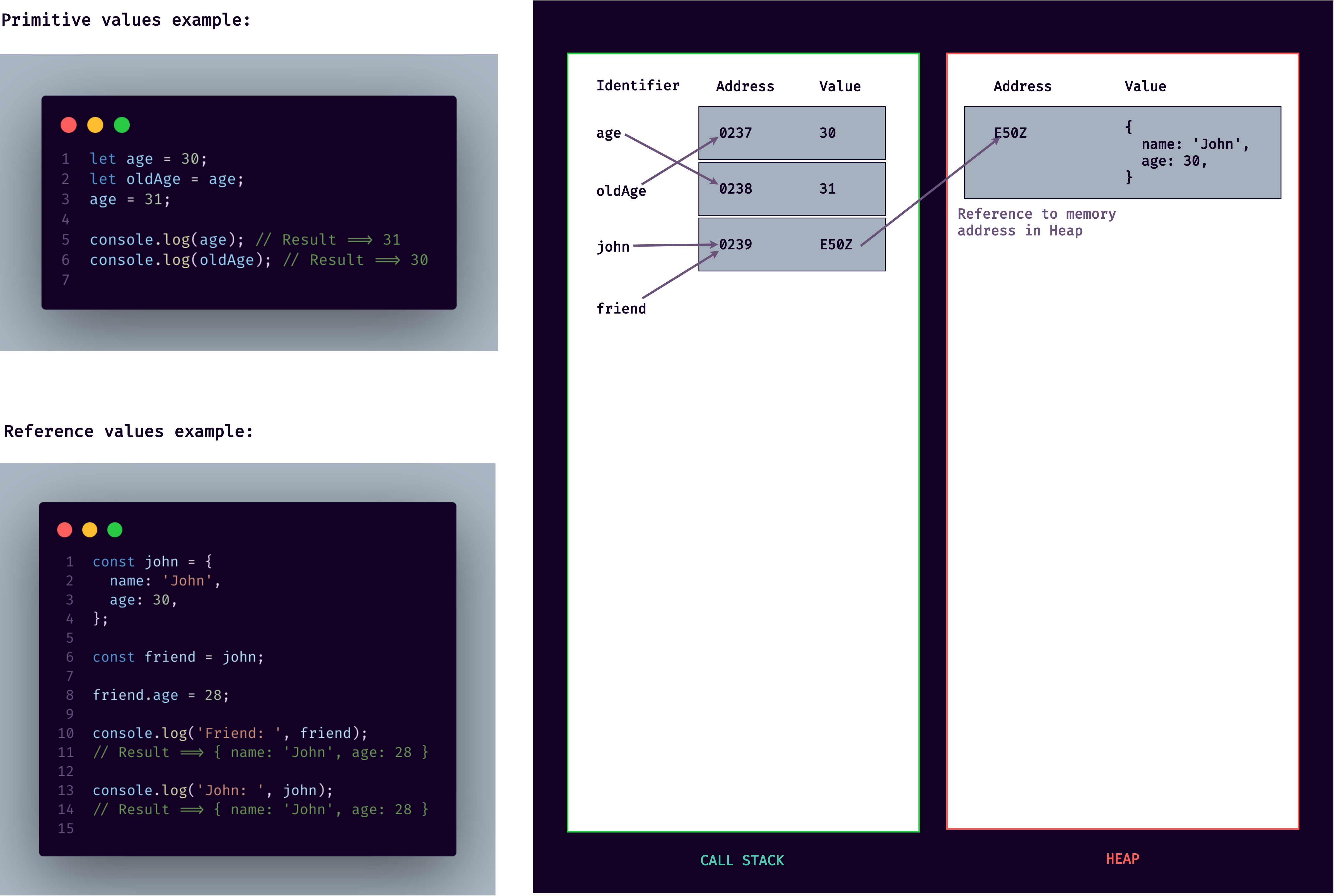Here comes the interesting part: now we're going to change a property in the `friend` object by setting `friend.age` to 28. What happens then is that the object is found in the heap, and the 30 is changed to 28.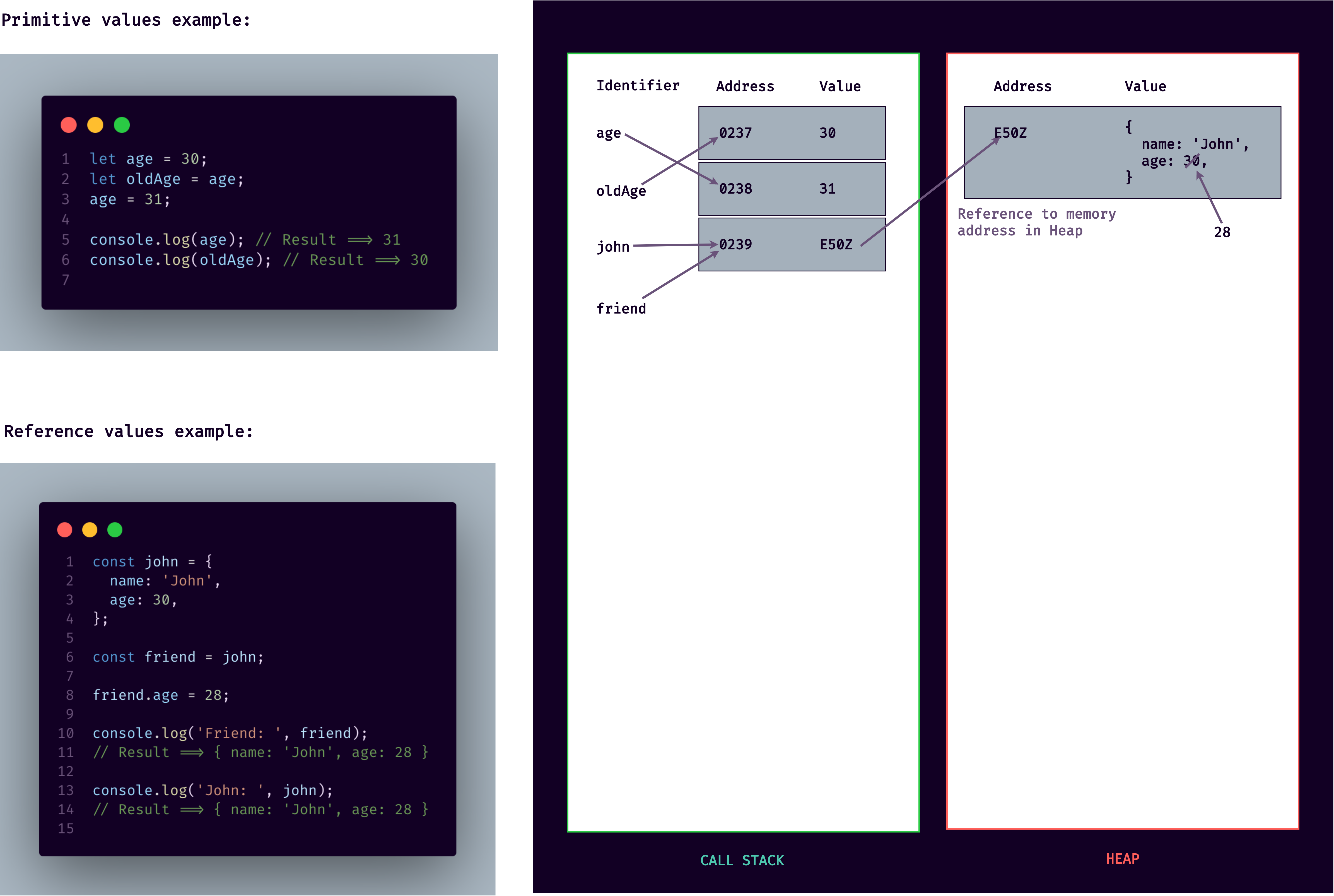Even though we defined the `friend` variable as a constant, we can actually still manipulate the object without problems. When we think about that, it makes sense because we're actually not changing the value in memory for the `friend` identifier. It is still E50Z, the reference to the object.

All we did was to change the value in the heap, and that's not a problem. It's a misconception that all variables declared with `const` are immutable. In fact, that is only true for primitive values, but not for reference values. So keep that in mind whenever you're working with `const`.

As we log the `friend` variable to the console, we get the age of 27, just as we said it before. But then, when we log the `john` object, we get that weird behavior that we could previously not explain and not understand. But with everything that we learned in this lecture, it actually now makes sense that in the `john` object, age is now also 27, even though we never changed `john.age` directly. And the reason for this, as we can see in the above image is the fact that `john` and `friend` point to the same object in the memory heap.

So whenever we change something in the object found in the heap, it will always be reflected in `friend` and `john`. `john` and `friend` are just two different identifiers pointing to the same value. And once again, that value is the memory address E50Z which points to the reference in the memory heap. One important implication of this is that whenever you think that you're copying an object, you're just creating a new variable that points to the same object.

This has huge implications for the way JavaScript works in practice. We will see that in the next lecture and throughout the course. There are actually ways around this, as we will also learn later. But in general, this is how reference values work in JavaScript.

So make sure to really understand this and the implications that this behavior has, even if that means that you have to read this lecture over again. Then once you really understand what happened here, in examples we had above, let's understand primitive values and reference values even better in practice in the next lecture.